# NCLEX : Dosage

## Example Questions

← Previous 1

### Example Question #1 : Pharmacology

The nurse is preparing to administer 250,000 units of medication to a patient. The ampule sent from the pharmacy contains 500,000 units/mL. How many mL should the nurse administer?

0.5 mL

0 mL

1.2 mL

2.5 mL

0.5 mL

Explanation:

The medication dose formula is as follows: Milliliters to be administered = (Dose to be administered)/(Available solution). Thus, the calculation for this problem is (250,000 units)/(500,000 units/mL) = 0.5 mL

### Example Question #1 : Nclex

The nurse is preparing to administer 100,000 units of medication to a patient. The ampule sent from the pharmacy contains 600,000 units/mL. How many mL should the nurse administer?

1.5 mL

2.4 mL

0 mL

2 mL

0 mL

Explanation:

The medication dose formula is as follows: Milliliters to be administered = (Dose to be administered)/(Available solution). Thus, the calculation for this problem is (100,000 units)/(600,000 units/mL) = 0 mL mL

### Example Question #2 : General Concepts

The nurse is preparing a bag of heparin sodium solution with a mix of 120,000 units/400 mL intravenously. At what rate should the nurse set the infusion pump if intending to deliver 1800 units/hr?

7.8 mL/hr

2.2 mL/hr

6.0 mL/hr

1.3 mL/hr

6.0 mL/hr

Explanation:

The required rate can be calculated as follows: (Delivered units/hr x mL)/units. Thus, the answer can be calculated as (1800 units/hr x 400 mL)/120,000 units = 6.0 mL/hr

### Example Question #4 : Pharmacology

Hayden is a 9-year-old boy who has been admitted to the emergency room with a fever. He weighs 71lbs. If the dosing guideline for acetaminophen is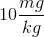at 4 hour intervals, how many milligrams of acetaminophen should the nurse administer?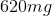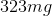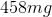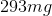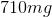Explanation:

First convert Hayden's weight from pounds to kilograms. This is done by dividing Hayden's weight by 2.2 because 1 pound is 2.2 kilograms.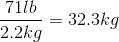Next set up the equation as follows: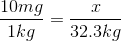Solve for: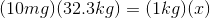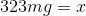### Example Question #11 : Pharmacology

An infant on his 14th day of life enters the emergency room. He are tachycardic, has poor skin turgor, and is crying without the formation of tears. The nurse knows that this child is showing symptoms of dehydration. The doctor orders fluid replacement of 544ml over 5 hours. What is the drip rate of the infusion?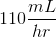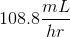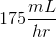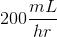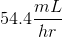Explanation:

Use the following formula to calculate drip rate: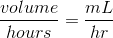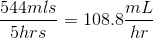### Example Question #1 : Dosage

An 11-month-old child weighing 8.6kg enters the emergency room with symptoms of a severe respiratory infection. Her physician suspects bacterial origin and prescribes Ampicillin. The guidelines state that Ampicillin is to be administered as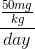in a dose q6 hours. Ampicillin comes in 250mg IV solutions of 50 milliliters. How many milliliters should the nurse administer for each dose?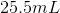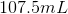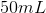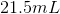Explanation:

The child's weight is already in kilograms. There is no need to change the unit of weight.

Set up the equation as follows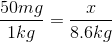Solve for: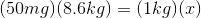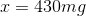By solving for, we have determined that the child is to receive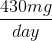.

Next, divide the dose by 4 because the dose is to be given every 6 hours.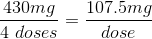By calculating this number, we have determined that the child should receive a dose of 107.5mg every six hours. If Ampicillin comes packaged with 250mg in 50mL, we can set up an equation to determine how many milliliters we need to reach 107.5mg.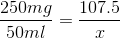Solve for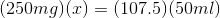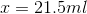### Example Question #1 : Dosage

Evelynn is a 3-year-old girl who has been bitten by her family's dog. She has had an incision and drainage (I&D) of her left hand to prevent infection and she has been prescribed cefazolin. The guidelines for cefazolin are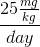. Evelynn weighs 42 lbs. How many milligrams of cefazolin should the nurse administer daily?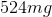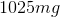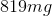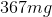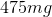Explanation:

First convert Evelynn's weight from pounds to kilograms. This is done by dividing Evelynn's weight by 2.2 because 1 pound is 2.2 kilograms.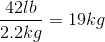Set up the equation as follows: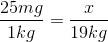Solve for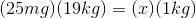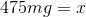### Example Question #11 : Nclex

The nurse must administer 1 liter of sodium chloride solution over 8 hours. What is the appropriate rate in milliliters per hour that the nurse must set on the IV pump?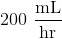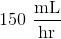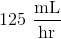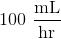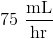Explanation:

In order to calculate the proper rate in milliliters per hour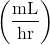, we must first convert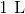to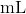, which is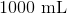. Then, we divide this volume in milliliters by 8 hours to get. This can be shown mathematically using dimensional analysis: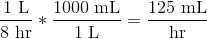### Example Question #1 : Dosage

The nurse prepares to administer naproxen to his patient. The physician has ordered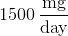in divided doses.

The medication label reads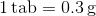. How many tablets equal the daily dose of this prescription?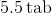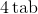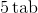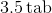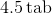Explanation:

First, convert the available dose to the dose ordered by the physician.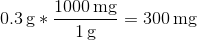Divide the prescribed daily dose by the dose in each tablet to get the number of tablets that equal the daily dose.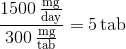### Example Question #11 : Nclex

The nurse administers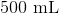of piperacillin to a patient with an infection. It must be delivered over 2 hours. The IV has a drop factor of 5. How many drops will fall per minute (rounded to the nearest tenth)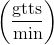?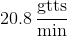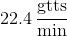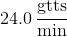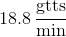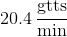Explanation:

The drops per minute equation is: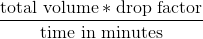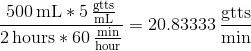Round to nearest tenth:← Previous 1

### All NCLEX Resources